# OliNo

Renewable Energy

## Integrallight LED downlighter recessed 1219.16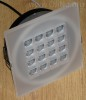presents an led based down lighter. It is their own design with 16 power leds. It has a nice semi transparent plate that is illuminated nicely when the light is on. The used powerunit is dimmable. An Eulumdat file is added in this article.

See this overview for a comparison with other light bulbs.

### Summary measurement data

parameter meas. result remark
Color temperature 3868 K Neutral white
Luminous intensity Iv 1985 Cd
Beam angle 30 deg
Power P 22.6 W
Power Factor 0.67 For every 1 kWh net power consumed, there has been 1.1 kVAhr for reactive power.
Luminous flux 657 lm
Luminous efficacy 29 lm/W
CRI_Ra 78 Color Rendering Index.
Coordinates chromaticity diagram x=0.3801 and y=0.3609
Fitting 230V
L x W x H external dimensions 145 x 145  x 75 mm External dimensions of the luminaire.
L x W luminous area 86 x 86 mm Dimensions of the luminous area (used in Eulumdat file). This is equal to the size of the area of the semi transparent flont plate that is filled with leds.
General remarks The ambient temperature during the whole set of measurements was 22-23.5 deg C.

Warm up effect: during the warm up time the light intensity decreases with 12 % and the color temperature increases with 5 %.

Voltage dependency: there is almost no dependency of  illuminance and power consumption on the voltage.

The Eulumdat file can be found via this link.

The light is well dimmable, see also the dimming test at the end.

Measurement report (PDF)### Eulumdat light diagram

An interesting graph is the light diagram, indicating the intensity in the C0-C180 and the C90-C270 plane. This light diagram below comes from the program Qlumedit, that extracts these diagrams from an Eulumdat file.The light diagram giving the radiation pattern.

It indicates the luminous intensity around the light bulb. The C0-C180 plane and the C90-C270 give the same result as the light bulb is almost symmetrical along the z-axis.

The light gives a focused beam.

The unit is Cd/1000lm, meaning the intensity in Cd assuming there would be 1000 lumen in the measured light bulb. This enables comparing different types of light bulbs.

### Illuminance Ev at 1 m distance, or luminous intensity Iv

Herewith the plot of the averaged luminous intensity Iv as a function of the inclination angle with the light bulb.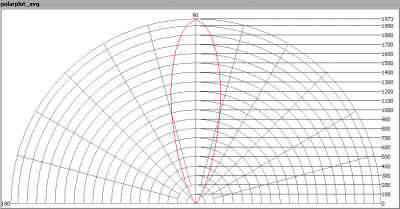The radiation pattern of the light bulb.

This radiation pattern is not the same as the one given earlier. This is because of the (small) differences of beam angles in the different planes. In this graph the luminous intensity is given in Cd.

These averaged values are used (later) to compute the lumen output.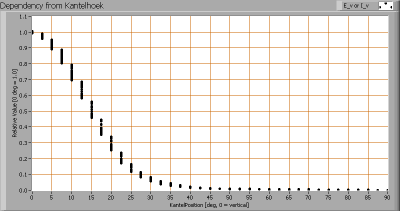Intensity data of every measured turn angle at each inclination angle.

This plot shows per inclination angle the intensity measurement results for each turn angle at that inclination angle.  There is little variation in intensity, for instance at 10 degrees inclination angle, the range in measured intensities was 70-80 % of the 0 degree inclination value.

As the average values of illumination per inclination angle will be used for further computations, there is no effect of any dispersion in intensity values on the further results.

When using the average values per inclination angle, the beam angle can be computed, being 30 degrees.

### Luminous flux

With the averaged illuminance data at 1 m distance, taken from the graph showing the averaged radiation pattern, it is possible to compute the luminous flux.

The result of this computation for this light spot is a luminous flux of 657 lm.

### Luminous efficacy

The luminous flux being 657 lm, and the power of the lightbulb being 22.6 W, yields a luminous efficacy of 29 lm/W.

A power factor of  0.67 means that for every 1 kWh net power consumed, a reactive component of 1.1 kVAr was needed.

 Light bulb voltage 230 V Light bulb current (luminaire) 146 mA Power P 22.6 W Apparent power S 33.6 VA Power factor 0.67

Of this light bulb the voltage across ad the resulting current through it are measured and graphed.Voltage across and current through the lightbulb
The current shows peaks at voltage polarity changes. This indicates the use of a switched mode power supply. The current has the same phase as the voltage, however it does not have a sine form (at all). Therefore the power factor is less than one.
Also the power spectrum of the current is determined.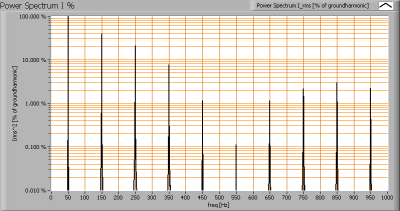The powerspectrum of the current through the light bulb.

There are higher harmonics apparent in the spectrum.

### Color temperature and Spectral power distribution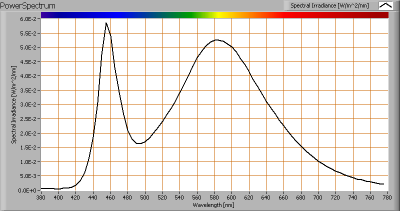The spectral power distribution of this light bulb.

The measured color temperature is about 3850 K which is neutral white.

This color temperature is measured straight underneath the light bulb. Below a graph showing the color temperature for different inclination angles.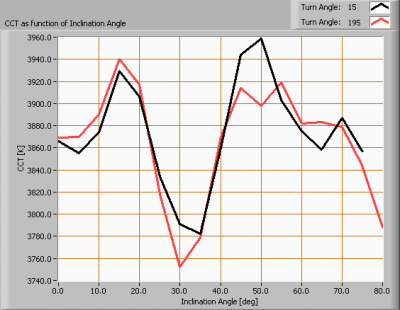Color temperature as a function of inclination angle.

The colortemperature is given for inclination angles up to 75 degrees since at larger inclination angles there is too little illumination.

The value stays within +/- 3 % of its initial value at 0 degrees inclination angle.

### Chromaticity diagram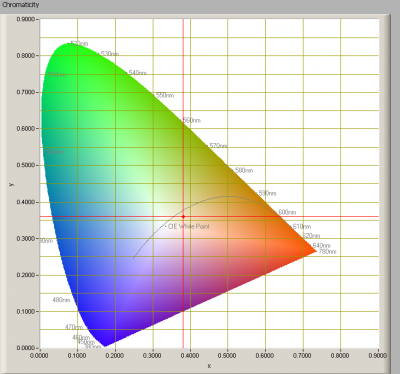The chromaticity space and the position of the lamp’s color coordinates in it.

The light coming from this lamp is at a distance from the Planckian Locus (the black path in the graph).

Its coordinates are x=0.3801 and y=0.3609.

### Color Rendering Index (CRI) or also Ra

Herewith the image showing the CRI as well as how well different colors are represented (rendered). The higher the number, the better the resemblance with the color when a black body radiator would have been used (the sun, or an incandescent lamp).

Each color has an index Rx, and the first 8 indexes (R1 .. R8) are averaged to compute the Ra which is equivalent to the CRI.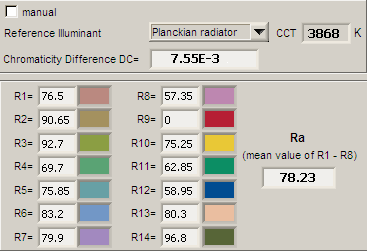CRI of the light of this lightbulb.

The value of 78 is lower than 80 which is considered a minimum value for indoor usage.

Note: the chromaticity difference is 0.008 indicates the distance to the Planckian Locus. Its value is higher than 0.0054, which means that the calculated CRI result is not meaningful.

### Voltage dependency

The dependency of a number of lamp parameters on the lamp voltage is determined. For this, the lamp voltage has been varied and its effect on the following lamp parameters measured: illuminance E_v [lx], color temperature CT or correlated color temperature CCT [K], the lamppower P [W] and the luminous efficacy [lm/W].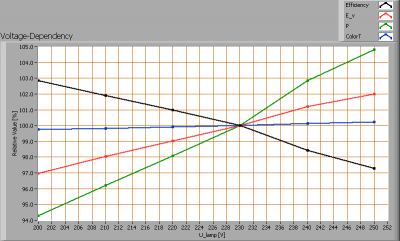Lamp voltage dependencies of certain light bulb parameters, where the value at 230 V is taken as 100 %.

The consumed power and the illuminance hardly vary when the voltage varies between 200 – 250 V. This variation is linear.

When the voltage at 230 V varies with + and – 5 V, then the illuminance varies with less than + and – 0.5 %, which will not be visible when the voltage changes abruptly.

### Warm up effects

After switch on of a cold lamp, the effect of heating up of the lamp is measured on illuminance E_v [lx], color temperature CT or correlated color temperature CCT [K], the lamppower P [W] and the luminous efficacy [lm/W].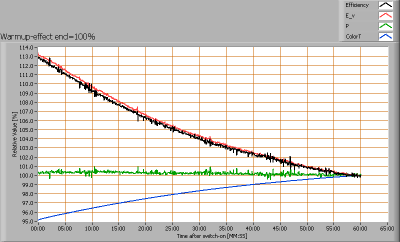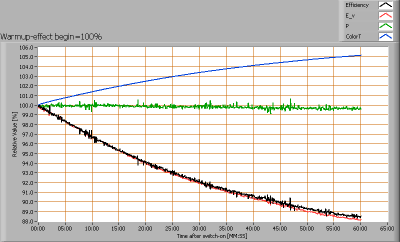Effect of warming up on different light bulb parameters. At top the 100 % level is put at begin, and at bottom at the end.

Warm up time is longer than 1 hour. During that time the light output decreased with 12 % and the color temperature increased 5 %.

### Dim ability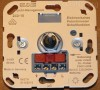The power unit makes dimming of the light possible. An electronic potmeter is used for that, see also photo. This electronic potmeter is attached via two specific wires to the power unit of the light bulb.

This potmeter is adjustable, see also the manual. The adjustment was done such that at the max dimming position the light output was minimal. At the min dimming position it was seen that the max lightput was present.

The potmeter has 36 positions, all of these are measured for light output, consumed power, luminous efficacy (computed by dividing light output by power) and color temperature.The influence of the mechanical dimmer position on diverse light bulb parameters

The light bulb is well dim-able. The mechanical range of the turn-knob that translates to effective dimming of the light, is from 65 % to 5 %. The light output then varies between 100 % and 5 %.

The consumed power drops even faster than the light output does (the leds give higher efficiency at lower temperatures) resulting in an increase of the efficacy. Finally at lower intensities (more dimming) the consumption of the power unit itself becomes more relevant and hence the efficacy drops.

The color temperature remains (relatively) constant.